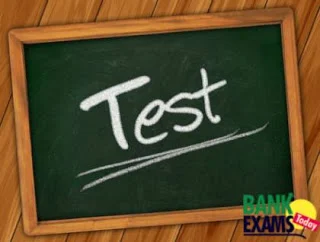New Student User - Use Code HELLO

# Mixed Aptitude Quiz -3 (For SBI PO 2016)#### Ques 1.

Train A leaves the station at a certain time and at a uniform speed After 7 hours Train B leaves the same station and moves in the same direction at a uniform speed of 152 km/hr. Train B catches up Train A in 9 hours. What is the speed of Train A (in km/hr.) ?

Solution :

==> (7X)/(152-X) = 9
==> X = 85.5 kmph

#### Ques 2.

The difference between simple interest on a certain sum at the rate of 16 p.c.p.a for one year and compound interest (compounded half yearly) on the same sum for the same period at the same rate is Rs. 235.52. What is the principal sum ?

Solution :

Compound Interest is compounded half yearly

So new rate = r/2 = 16/2=8

new time =2*t = 2*1 = 2

C.I. rate of interest = 16.64

S.I rate of interest = 16

difference = 235.52

0.64----- 235.52
100-------?

Sum = 36800

#### Ques 3.

20 men can do a work in 15 days. One man start then work and after everyday one more man joins the work. How many day needs to complete the work ?

Solution :

n(n+1)/2 = 20*15

N = days = 24

#### Ques 4.

In an Entrance Examination Rini scored 56 per cent marks. Smita scored 92 per cent marks and Rina scored 634 marks. The maximum marks of the examination are 875. What are the average marks scored by all the three girls together?

Solution :

56/100x875 =490

92/100x875 = 805

Average =490+805+643/3 =1929/3 =643

#### Ques 5.

I. 168x^2 − 70x + 7 = 0
II. 36y^2 − 21y + 3 = 0

Solution :

X = 1/4 , 1/6

Y = 1/4, 1/3

X<=Y

#### Ques 6.

Some balls are distributed among 4 boys M, N, O and P such that the respective ratio of number of balls received by M and N is 7 : 10. Moreover P received 8 balls more than M and O received 4 balls more than N. If P received 4 balls less than N then how many balls did O receive ?

Solution :

M: N = 7:10

M = 7X ; N =10X

P = 7X+4
O = 10X+4

==> 7X+8+4=10X
==>X = 4

O = 40+4=44

#### Ques 7.

Six-eleventh of a number is equal to twenty two per cent of second number. Second number is equal to the one-fourth of third number. The value of the third number is 2400. What is the 45% of first number?

Solution :

N2 = 2400/4 = 600
6/11 N1 = 22% N2
N1 = 600*22/100 * 11/6 = 242

242*45% = 108.9

#### Ques 8.

The ratio between present ages of M and N is 4 : 5 respectively. The ratio between M’s age 8 years ago and N’s age 2 years hence is 4 : 7 respectively. What is the respective ratio between M’sage 4 years hence and N’s age 8 years ago ?

Solution :

4x-8/5x+2 = 4/7
==>X= 8

M 's age 4 years hence and N's age 8 years ago =36: 32 = 9:8

#### Ques 9.

What will be the difference between the simple interest and compound interest earned on a sum of Rs. 985 @ 14 p.c.p.a. at the end of two years?

Solution :

Difference = P * (14/100)^2

==> Difference = 985(14/100^2)=19.2

#### Ques 10.

The average marks in English subject of a class of 24 students is 56. If the marks of three students were misread as 44, 45 and 61 of the actual marks 48, 59 and 67 respectively, then what would be the correct average?

Solution :

Difference = (48+59+67)-(44+45+61)=24

==>Increased Average =Difference/ total members=24/24 = 1
==>previous Average + increased average =56 + 1 = 57